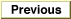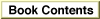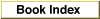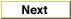Legacy DocumentImportant: The information in this document is obsolete and should not be used for new development.

Inside Macintosh: QuickDraw GX Environment and Utilities /
Chapter 8 - QuickDraw GX Mathematics / Using QuickDraw GX Mathematics

Performing Vector Operations

You can use the VectorMultiply function to obtain the dot product of two vectors with 64-bit accuracy. The function takes six parameters: the first parameter specifies the number of long numbers to multiply, and the third and fifth parameters specify the step size to use when walking the arrays to which the second and fourth parameters point.

For example:

VectorMultiply(4,a,1,b,2,&c) sets the wide number pointed to by the parameter c to the following value:

a * b +a  * b +a * b + a * b

If the count is negative, the sign of the terms in the dot product are alternated.

VectorMultiply(-4,a,1,b,2,&c) sets the wide parameter c to the following value and the result is returned in c:

a * b - a * b + a * b - a * b

You can also use VectorMultiply to determine the cross-product of a pair of vectors, as in Listing 8-1.

Listing 8-1 Calculating a cross-product with VectorMultiply

gxPoint *CrossProduct(const gxPoint *a, gxPoint *b, )
{
wide temp;
WideShift(VectorMultiply(-2, &a->x, 1, &b->y, -1, &temp), 16);
}
You can also use VectorMultiply to work with mappings. Listing 8-2 is a sample function that applies a mapping to a single point.

Listing 8-2 Applying a mapping to one point

gxPoint *MapPoint(const gxMapping *map, gxPoint *pt)
{
fixed temp = { 0, 0, fixed1 };
*(gxPoint *)temp = *pt;
wide dot;
fixed p = WideShift(VectorMultiply(3, temp, 1, &map,
3, &dot), 30);
pt->x = WideDivide(VectorMultiply(3, temp, 1, &map,
3, &dot), p, nil);
pt->y = WideDivide(VectorMultiply(3, temp, 1, &map,
3, &dot), p, nil);
return pt;
}
The VectorMultiply function is described on page 8-54. Functions that perform vector operations are described in the section "Vector Operations" beginning on page 8-54.